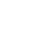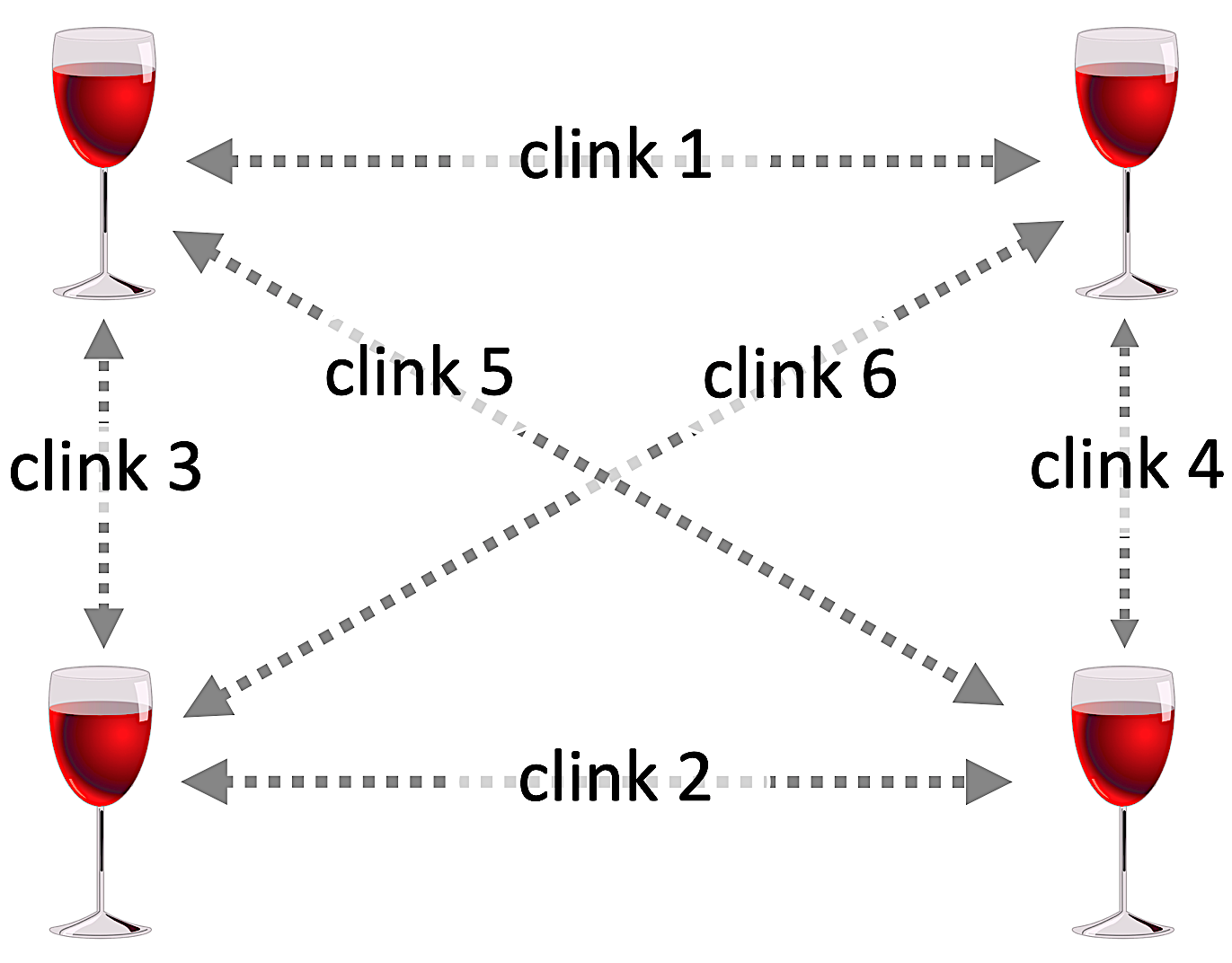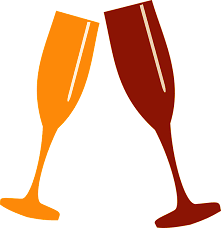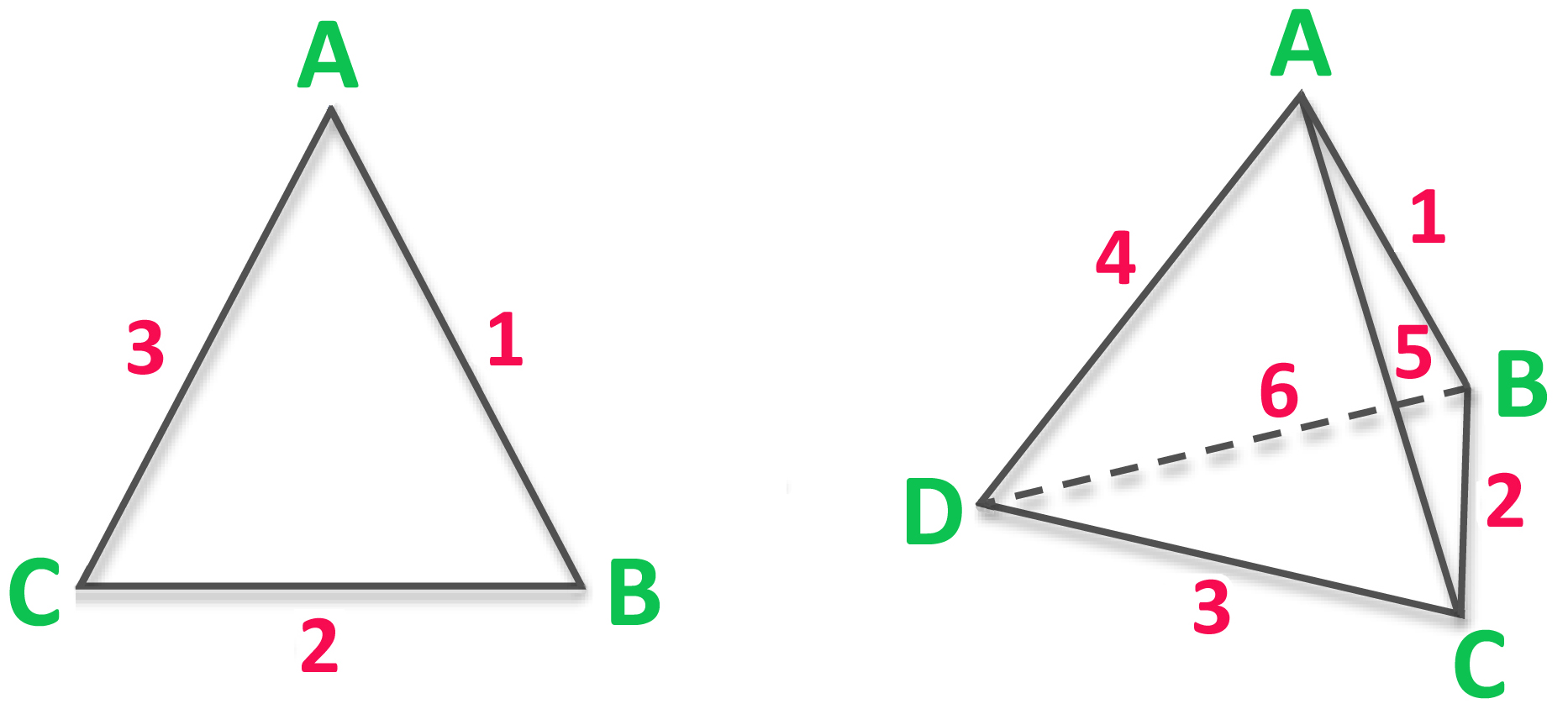This convivial experiment is a neat way to explain Euler's Law#### Context

1. The model of a tetrahedron can help to explain the benefits of thinking in fours.
2. Some find it helpful and fascinating, but others are intimidated by geometrical figures.
3. Also, whereas Euclidean geometry implies a timeless Utopia, this experiment works in social time-space.
4. It can be an icebreaker on many levels.

#### ActionsThis can work well in front of a large, mixed group of colleagues

1. Select at least 4 volunteers, preferably strangers representing different departments, interests, types or backgrounds.
2. Give each volunteer a wine or beer glass, then ceremoniously fill each with a festive beverage.
3. Ask an accountant/s to estimate the per capita cost of this experiment.
4. Ask 2 of the 4 volunteers to clink their glasses together, then point out that:
• a) You still have the original investments (2 employees + 2 intact drinks)
• b) You may now have an additional level of synergy (e.g. rapport) between the 2 investments.
• c) Even if you can't see the additional asset yet (represented by the 'clink') it cost nothing to arrange.
• d) Emphasise that this is not a sales pitch for alcohol. It exemplifies how relational thinking can create a free gift.
• A plus B can give you C (which you didn't have before)...yet you still have A and B.
5. Point out that, at this stage, you now still have more (tangible) assets than synergies, so we need a profit.
6. Ask a third volunteer to join the first pair. Ask the audience how many clinks the toasting trio will make.
7. Wait until everyone agrees the answer, then get the drinkers to confirm the theory by clinking one another.
8. Point out that the company now has an additional 2 free gifts (assets) and ask whether we are in profit....
9. Obviously, we have broken even (3 existing assets and 3 new ones). Ask how many clinks one more drinker will make.
10. Wait until the audience agrees a number. Ask the fourth drinker to step forward....test out the theory.
11. Point out that we all heard 6 clinks from 4 drinkers and that we are now 'in profit'.
12. Another way to explain this is that a quartet makes 6 times more synergy than a duet....etc. etc.

#### Euler's Law

The good news is that, if we can transcend the flatland of unit-based money, we can create new opportunities that exist when the logic of (re)combination is scaled up to a three-dimensional level. A helpful starting point is Euler's Law, of 1751, which demonstrates why it will be auspicious to design future business models around collaborative clusters of at least four players, or combinations of at least four assets.

1. Euler’s law (1752) derives from polygons, but the same logic pertains to the 'relationships' between 'things'.

(i.e. V+F=E+2). Euler showed that, in any polygon, the number of vertices (V) plus the number of Faces (F) equals the number of Edges (E) plus two.• Euler's Law (1751) states that V + F = E + 2 where -
• V represents the number of vertices
• F represents the number of faces
• E represents the number of edges

See further notes on intrinsic abundances
See some useful equations